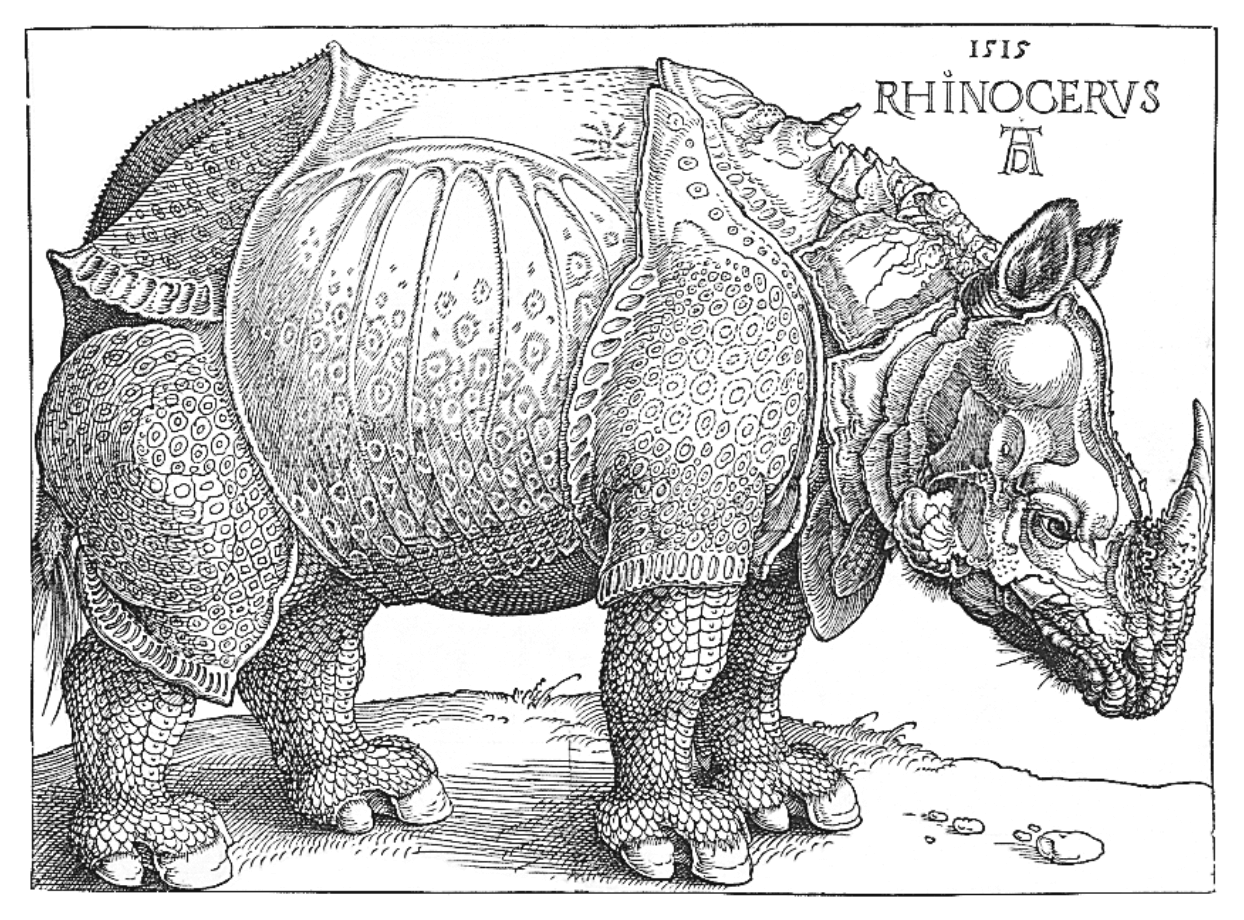# Hugo-Tufte Features

Fancy Subtitle

Contents

This is a quick demonstration post. It serves as an example of the features of this theme. One of them is $$\LaTeX$$ via MathJax.

### Inline

Some inline math: This is a margin note.

$e^{i \pi} = -1$ and $$\sqrt{-1} = i$$ and $$a_2 = 3$$.

### Display

And display math using escaped brackets \$: This is a sidenote! \[ – \cdot_H – \colon B(G,H) \times B(H, K) \to B(G, K), \quad ([X], [Y]) \mapsto [X \times_H Y].$

### Environments

Currently, certain $\LaTeX$ environments need to be escaped so that the markdown processor does not override MathJax. Currently, display environments should be enclosed in <p> tags and blank lines. For instance:

is produced from

<p>
\begin{align*}
\mu(A) &= \iint_{I^2} \chi_A (x,y) \ d(x,y)
= \int_I \left( \int_I  \chi_A (x,y) \ dx\right) dy
= \int_I 0 \ dy= 0 \quad \text{and} \\
\mu(A) &=\iint_{I^2}  \chi_A (x,y) \ d(x,y)
= \int_I \left(  \int_I \chi_A (x,y) \ dy \right) dx
=\int_I dx = 1,
\end{align*}
</p>


### Blockquotes

Some blockquotes. But first, we try to manually cite via This is between cite tags and has math: $$e^x$$

This is a blockquote with two paragraphs, that employs the theme’s blockquote shortcode. Lorem ipsum dolor sit amet, consectetuer adipiscing elit. Aliquam hendrerit mi posuere lectus. Vestibulum enim wisi, viverra nec, fringilla in, laoreet vitae, risus.

Donec sit amet nisl. Aliquam semper ipsum sit amet velit. Suspendisse id sem consectetuer libero luctus adipiscing. Vestibulum enim wisi, viverra nec, fringilla in, laoreet vitae, risus.

### New thoughts

Sometimes a new thought distinguishes a section, as here. There are currently two ways to create one. One way is with raw HTML such as: <span class="newthought">...</span>". The theme also provides the newthought shortcode.

### Code

As an example of some inline code: go test -v -short. And this is some block-code:

package main

import "log"

func add(x int, y int) int {
log.Println("We are going to take the sum of two numbers, and leave a long comment.")
return x + y
}

func main() {
log.Println(y)
}


### Figure

Below we have an example of a regular width figure.

And now we exhibit a margin figure.The image title. This is the image caption. Image attribution

Below is a full-width figure.